×

Search anything:

# xargs command in Linux

#### Linux Software EngineeringWe use xargs to build an execution pipeline whereby we can send input to another command that does not accept piped input such as echo, mkdir, etc. In this article, we have learned the uses of xargs using various examples.

1. Introduction.
2. Syntax.
3. Usage.
4. Summary.
5. References.

## Introduction.

We use the xargs command to build an execution pipeline from the standard input. We use it in cases where we want to send input to a command that does not accept piped input. For example, commands such as rm, echo, mkdir cannot accept standard input as its arguments.

## Syntax.

We write xargs commands using the following syntax:

``````\$ xargs [OPTION]... COMMAND [INITIAL-ARGS]...
``````To pass the standard output of a command to xargs we pipe it as follows:

``````\$ command1 | xargs command2
``````

## Usage.

1. To get started, let us execute the command without argument:
``````\$ xargs
``````

After we execute the above command, we can type input, and when done press CTRL+d. After this all the text typed will be printed out:

``````\$ xargs
xargs
builds
an execution
pipeline
# press CTRL+d
``````1. The common usage of this command is with the find command. That is, after we locate a file, we perform operations on it. For example, let's find a file and move it or change its attributes.
``````\$ find *.pdf | xargs chmod 600
``````

Here we find all files with a .pdf file extension and change their file permissions.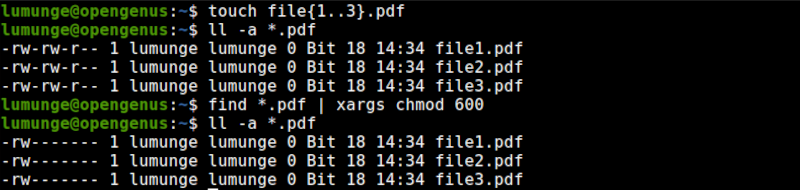1. Now, let's use the xargs command with the grep and find commands. Here we first find a file then search for a string in the file.
``````\$ find /var/log -type f -name "*.log" | xargs grep "error"
``````

In this example, we find all .log files in the /var/log directory and search for the text error in the files.

1. The -a option used with xargs command is used to read from a file as shown below.
``````\$ xargs -a [file name]
``````
1. From the previous example, xargs will do its part even if there is no content in the file testXargs. In such cases, it executes the /bin/echo which prints a new line. We can prevent this by using the -r option:
``````\$ xargs -r -a testXargs
``````1. Let's try to pipe echo command output to the mkdir command. That is echo text and use the echoed text as names for new directories we create using the mkdir command:
``````\$ echo "Dir1 Dir2" | xargs mkdir
``````

In this example, we create two directories, Dir1 and Dir2.1. xargs command is not limited to executing a single command, we can also execute multiple commands by using the -I option. As an example, let us create two directories and change their permissions:
``````\$ echo "Dir1 Dir2" | xargs -I % sh -c 'mkdir %; chmod 600 %'
``````

First, we echo Dir1 Dir2 which are separated by white space, we then create two directories with the names of the echoed text and finally change the permissions of the directories.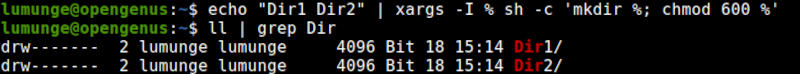1. From the examples, no output is printed after the command executes, in some cases, we may want to examine the command that was executed for debugging purposes. In such situations, we use the -t option which prints out the command.
``````\$ echo "Dir1 Dir2" | xargs -t mkdir
``````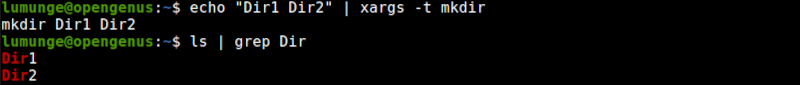Another example: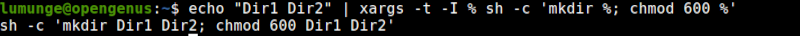In this example sh calls the shell interpreter and we pass the -c option which states that sh should execute the program as interpreted by
the program.

1. In Linux, some commands are irreversible, that is, once executed we cannot go back, for example, if we remove a file or directory, we cannot undo this. In such cases, we use prompts, these will ensure that we are prompted before we act. We use the -p option with the xargs command as follows:
``````\$ echo "Dir1 Dir2" | xargs -p mkdir
``````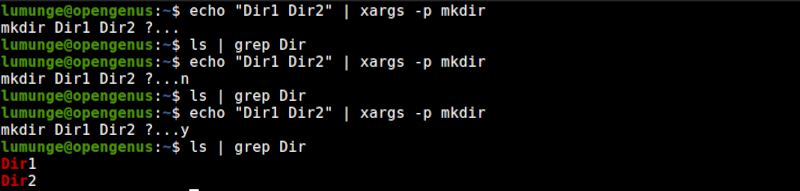1. Sometimes we want to pass at most n arguments to the xargs command. For example,
``````\$ echo "1 2 3 4 5 6 7" | xargs -n4
``````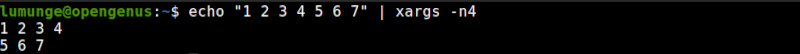We can also use it with the -p option to get prompted as follows:

``````\$ echo "1 2 3 4 5 6 7" | xargs -n4 -p
``````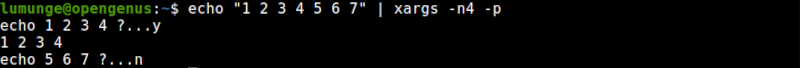1. The xargs delimits arguments using blank space. We can change this using the -d option as follows:
``````\$ echo "Dir1*Dir2*Dir3*" | xargs -d\* mkdir
``````In this case, we use * character as the delimiter. This is useful in cases where we are performing operations using text with a certain format. For example CSV files.

1. The xargs command is also used to remove whitespace from files or strings with whitespace:
``````\$ ping -c 1 [IP] | xargs
``````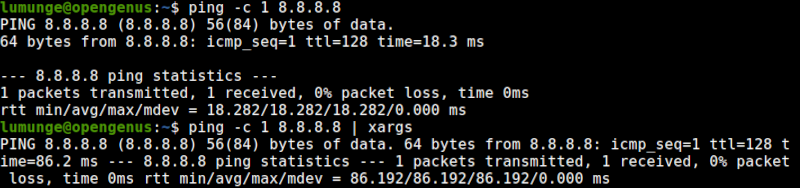1. Usually the OS will have limits on how the xargs command operates. To show these limits we execute the xargs command with the --show-limits
``````\$ xargs --show-limits
``````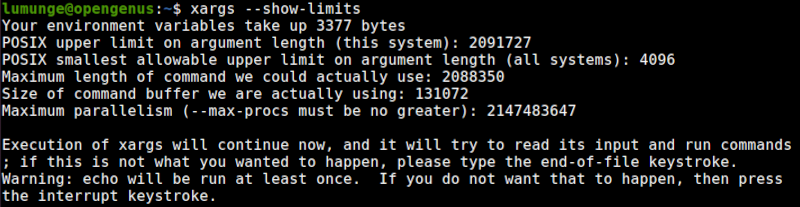## Summary.

We use xargs to build an execution pipeline whereby we can send input to another command that does not accept piped input such as echo, mkdir, etc.
In this article, we have learned the uses of xargs using various examples.

For a comprehensive guide on the xargs command, we can checkout its manual by executing the command \$ man xargs.

#### Erick Lumunge

Erick is a passionate programmer with a computer science background who loves to learn about and use code to impact lives positively.

xargs command in Linux